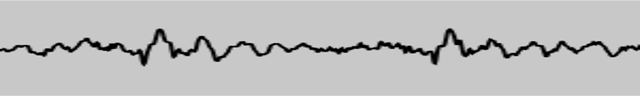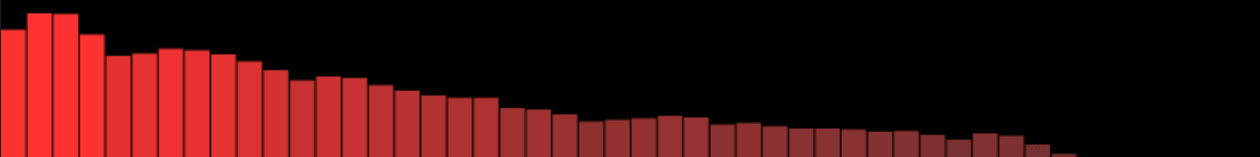# 基于 Web Audio API 实现音频可视化效果

## 基本概念

js

``````var audioCtx = new (window.AudioContext || window.webkitAudioContext)();
var analyser = audioCtx.createAnalyser();
``````

js

``````source = audioCtx.createMediaStreamSource(stream);
source.connect(analyser);
analyser.connect(distortion);
// etc.
``````

js

``````analyser.fftSize = 2048;
var bufferLength = analyser.frequencyBinCount;
var dataArray = new Uint8Array(bufferLength);
``````

js

``````analyser.getByteTimeDomainData(dataArray);
``````

## 创建一个波形/示波器

js

``````analyser.fftSize = 2048;
var bufferLength = analyser.fftSize;
var dataArray = new Uint8Array(bufferLength);
``````

js

``````canvasCtx.clearRect(0, 0, WIDTH, HEIGHT);
``````

js

``````function draw() {
``````

js

``````drawVisual = requestAnimationFrame(draw);
``````

js

``````analyser.getByteTimeDomainData(dataArray);
``````

js

``````canvasCtx.fillStyle = "rgb(200, 200, 200)";
canvasCtx.fillRect(0, 0, WIDTH, HEIGHT);
``````

js

``````canvasCtx.lineWidth = 2;
canvasCtx.strokeStyle = "rgb(0, 0, 0)";

canvasCtx.beginPath();
``````

js

``````var sliceWidth = (WIDTH * 1.0) / bufferLength;
var x = 0;
``````

js

``````for (var i = 0; i < bufferLength; i++) {
var v = dataArray[i] / 128.0;
var y = (v * HEIGHT) / 2;

if (i === 0) {
canvasCtx.moveTo(x, y);
} else {
canvasCtx.lineTo(x, y);
}

x += sliceWidth;
}
``````

js

``````      canvasCtx.lineTo(canvas.width, canvas.height/2);
canvasCtx.stroke();
};
``````

js

``````draw();
``````## 创建一个频率条形图

js

``````analyser.fftSize = 256;
var bufferLength = analyser.frequencyBinCount;
console.log(bufferLength);
var dataArray = new Uint8Array(bufferLength);

canvasCtx.clearRect(0, 0, WIDTH, HEIGHT);
``````

js

``````    function draw() {
drawVisual = requestAnimationFrame(draw);

analyser.getByteFrequencyData(dataArray);

canvasCtx.fillStyle = 'rgb(0, 0, 0)';
canvasCtx.fillRect(0, 0, WIDTH, HEIGHT);
``````

js

``````var barWidth = (WIDTH / bufferLength) * 2.5;
var barHeight;
var x = 0;
``````

js

``````      for(var i = 0; i < bufferLength; i++) {
barHeight = dataArray[i]/2;

canvasCtx.fillStyle = 'rgb(' + (barHeight+100) + ',50,50)';
canvasCtx.fillRect(x,HEIGHT-barHeight/2,barWidth,barHeight);

x += barWidth + 1;
}
};
``````

js

``````draw();
``````